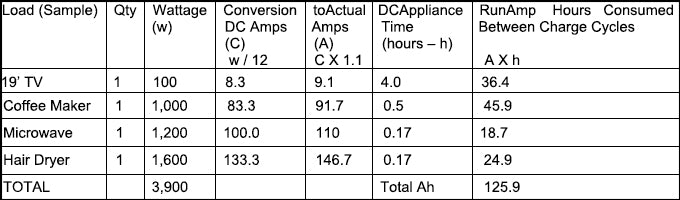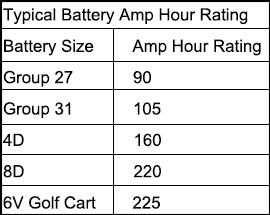# Can I know more about Inverter and battery bank selection for specific load?Choosing the right Inverter

When sizing your inverter, calculate the total wattage required at any one time and choose the inverter with a slightly higher power output. (Start up surge should be considered for compressive loads.)

1. Converting AC amps to Watts: AC amps x 120 Volts = Watts

2. Convert Watts to Actual DC amps: (Watts / 12) x 1.1 = actual DC amps

3. amp hours consumed between charge cycles:run-time of appliance (hours) X actual DC amps = amp hours consumed

4. Number of batteries required:(Total amp hours consumed X 2*) / amp hour rating of battery = Number of batteries required*Since deep cycle batteries should only be discharged to 50% total capacity, the total amp hours consumed between charge cycles should be multiplied by 2.

Example:

amp hours consumed between charge cycle = 126

amp hour rating of battery = 90 (Group 27)

(126X2) / 90 = 2.8

(Round up to the nearest whole number.)
Therefore, the minimum number of batteries required is three with the above values.### can you use this unit with it plugged into a cigarette lighter or does it have to be wired directly to the battery?

For any power inverter which is higher than 400Watt or more has to be wired straight to battery.

No it shouldn't.